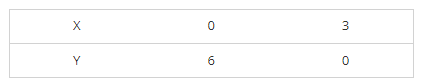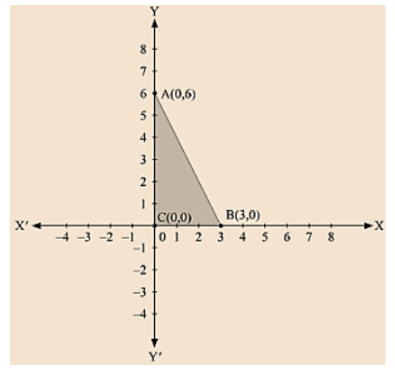# Draw the graph of the equation 2x + y = 6. Shade the region bounded

Question:

Draw the graph of the equation 2x + y = 6. Shade the region bounded by the graph and the coordinate axes. Also, find the area of the shaded region.

Solution:

We are given, 2x + y = 6

We get, y = 6 - 2x

Now, substituting x = 0 in y = 6 - 2x,

we get y = 6

Substituting x = 3 in y = 6 - 2x, we get y = 0

Thus, we have the following table exhibiting the abscissa and ordinates of points on the line represented by the given equationThe region bounded by the graph is ABC which forms a triangle.

AC at y axis is the base of triangle having AC = 6 units on y axis.

BC at x axis is the height of triangle having BC = 3 units on x axis.

Therefore, Area of triangle ABC, say A is given by A = (Base x Height)/2

A = (AC × BC)/2

A = (6 × 3)/2

A = 9 sq. units